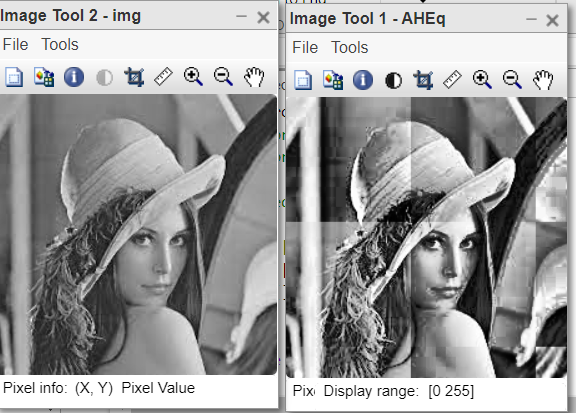Open In App

# Adaptive Histogram Equalization in Image Processing Using MATLAB

Histogram Equalization is a mathematical technique to widen the dynamic range of the histogram. Sometimes the histogram is spanned over a short range, by equalization the span of the histogram is widened. In digital image processing, the contrast of an image is enhanced using this very technique.

Adaptive Histogram Equalization: Adaptive histogram equalization is a digital image processing technique used to enhance the contrast of images. It differs from normal histogram equalization in the respect that the adaptive method enhances the contrast locally. It divides the image into distinct blocks and computes histogram equalization for each section. Thus, AHE computes many histograms, each corresponding to a distinct section of the image. It enhances the local contrast and definitions of edges in all distinct regions of the image.

• It computes the HE of distinct sections of the image.
• It preserves the edges in distinct regions of the image.
• It enhances the contrast locally.

AHE overamplifies the noise in relatively homogeneous regions of an image. To prevent this a variant of adaptive histogram equalization called contrast limited adaptive histogram equalization (CLAHE) is used.

Function Used:

• size( ) is in-built function used to get the size of image.
• rgb2gray( ) is in-built function used to convert RGB image into grayscale image.
• zeros(row, col) is in-built function used to create row*col matrix of zeros.
• unit8( ) is in-built function used to convert double value into integer format.
• blockproc( ) is in-built function used to apply HE function to distinct sections of the image.
• length( ) is in-built function used to find the size of list.
• imtool( ) is in-built function used to display image.
• pause( ) is in-built function used to pause the system to execute next statements.

## Matlab

 `% MATLAB code for Histogram equalisation``% function to return resultant``% image: Apply on single channel only.``function` `res_img=myhistEq(img)``Freq=zeros(1,256);``[x,y,z]=size(img);`` ` `% Convert into grayscale if ``% image is coloured.``if``(z==3)``    ``img=rgb2gray(img);``end`` ` `% Calculate frequency of each``% intensity value.``for` `i=1:x``    ``for` `j=1:y``        ``Freq(img(i,j)+1)=Freq(img(i,j)+1)+1;``    ``end``end`` ` `% Calculate PDF for each intensity value.``PDF=zeros(1,256);``Total=x*y;``for` `i=1:256``    ``PDF(i)=Freq(i)/Total;``end`` ` `% Calculate the CDF for each intensity value.``CDF=zeros(1,256);``CDF(1)=PDF(1);``for` `i=2:256``    ``CDF(i)=CDF(i-1)+PDF(i);``end`` ` `% Multiply by Maximum intensity value``% and round off the result.``Result=zeros(1,256);``for` `i=1:256``    ``Result(i)=uint8(CDF(i)*(255));``end`` ` `% Compute the new image.``new_img=zeros(size(img));``for` `i=1:x``    ``for` `j=1:y``        ``new_img(i,j)=Result(img(i,j)+1);``    ``end``end``res_img=new_img;``end`` ` `%%%%% UTILITY CODE %%%%%%%%``fun=@(block_struct)myhisteq(block_struct.data);`` ` `%blockproc() is block processing function.``%it applies normal HE on distinct block of``%defines sizes [m n]`` ` `list=[``"hat_lady.jfif"``];``for` `i=1:length(list)``    ``img=imread(list(i));``    ``AHEq=blockproc(img,[100 100], fun);``    ``%AHEq=blockproc(img,[200 200], fun);``    ``%AHEq=blockproc(img,[250 200], fun);``    ``%HEq=myhisteq(img);``    ``%imtool(HEq,[]);``    ``imtool(AHEq,[]);``    ``imtool(img,[]);``    ``pause(10);``    ``imtool close all;`

Output:AHE is better than ordinary HE when the image has extremely dark or bright spots. But AHE tends to overamplify the contrast in near-constant regions of the image since the histogram in such regions is highly concentrated. As a result, AHE may cause noise to be amplified in the near-constant region.